# Chapter 11 Lab 9 - 09/12/2021

In this lecture we will introduce the concepts of leverage, studentized residuals and influential observations. Finally, we will use the residuals to check the assumptions behind the linear regression model. For the theoretical details of these topics, please refer to lecture slides.

We will use the data contained in the file logret.csv referred to the log returns of the following assets:

• The Boeing Company (BA),
• Caterpillar Inc. (CAT),
• Cisco Systems Inc. (CSCO),
• Nike Inc. (NKE),
• The Walt Disney Company (DIS)
• the Dow Jones Industrial Average index (DJI).

logret = read.csv("./files/logret.csv")
str(logret)
## 'data.frame':    1258 obs. of  6 variables:
##  $BA : num -0.02662 0.03486 0.00429 -0.02535 0.01833 ... ##$ CAT : num  -0.01279 0.00467 0.00675 0.00116 -0.0059 ...
##  $CSCO: num 0.000985 -0.000492 0.006873 0.001955 0.023644 ... ##$ NKE : num  -0.01063 0.000954 0.001905 0.010412 0.001694 ...
##  $DIS : num -0.004129 0.000394 0.000197 -0.004143 0.000197 ... ##$ DJI : num  -0.00415 0.00462 0.00601 0.00128 0.0014 ...

We estimate first of all the full regression multiple model with DJI being the dependent variable and the remaining variables as predictors:

mod1 = lm(DJI ~ ., data=logret)

The code ~ . specifies that all the variables in the data frame, except DJI, should be included as regressors.

From the summary output

summary(mod1)
##
## Call:
## lm(formula = DJI ~ ., data = logret)
##
## Residuals:
##        Min         1Q     Median         3Q        Max
## -0.0161881 -0.0022201 -0.0000164  0.0021291  0.0143476
##
## Coefficients:
##               Estimate Std. Error t value Pr(>|t|)
## (Intercept) -4.066e-05  9.917e-05   -0.41    0.682
## BA           1.628e-01  8.804e-03   18.50   <2e-16 ***
## CAT          1.531e-01  7.987e-03   19.17   <2e-16 ***
## CSCO         1.225e-01  8.582e-03   14.27   <2e-16 ***
## NKE          9.944e-02  8.120e-03   12.25   <2e-16 ***
## DIS          1.655e-01  1.021e-02   16.20   <2e-16 ***
## ---
## Signif. codes:  0 '***' 0.001 '**' 0.01 '*' 0.05 '.' 0.1 ' ' 1
##
## Residual standard error: 0.003501 on 1252 degrees of freedom
## Multiple R-squared:  0.7668, Adjusted R-squared:  0.7659
## F-statistic: 823.3 on 5 and 1252 DF,  p-value: < 2.2e-16

we can conclude that all the $$\beta$$ coefficients are significantly different from zero and none of the predictors should be removed. The goodness of fit is quite high.

## 11.1 Problematic observations

Problematic observations are defined according to three concepts: leverage, residual and influence (described in the following sections). In the plots of Figure 11.1 we have a bunch of black points and a red point that could be problematic. The black points are used to estimate the black line; the red line instead is estimated by use the black AND the red points. The following 4 situations are represented:

• top-left: the red point has a high residual value, a small leverage value and a low influence (in fact the red and black estimated regression line are pretty close);
• top-right: the red point has a high residual, a high leverage and it’s also highly influential (in fact the red line is quite different from the black line, estimated without the red point);
• bottom-left: the red point has a high leverage but a small residual and a small influence (the black and red line basically coincide);
• bottom-right: the red point has a high residual, a small leverage but a high influence (the two lines differ).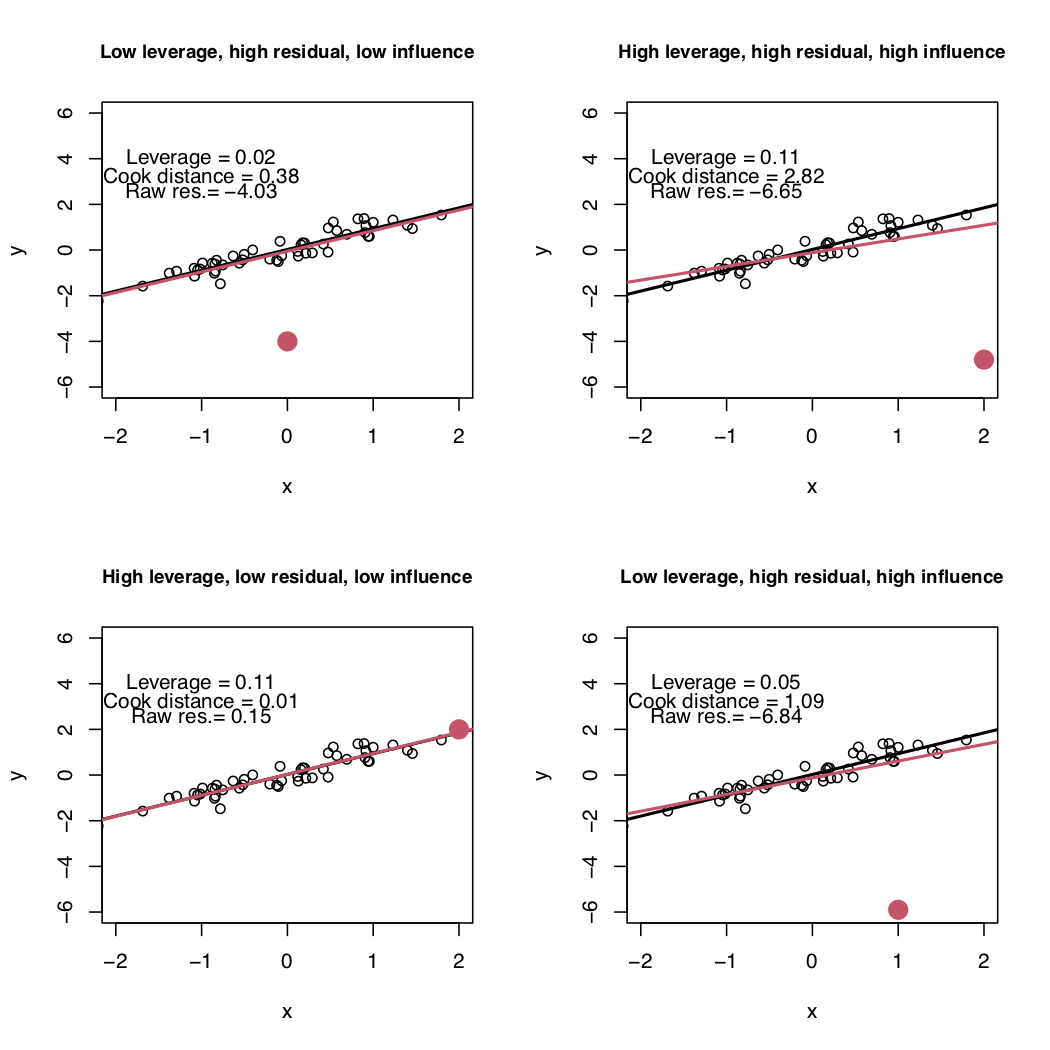Figure 11.1: Simple linear regression model: four possible situations with respect to leverage, residual and influence.

### 11.1.1 Leverage

A high leverage point is an observation with a value of the independent variables $$x$$ far from the mean $$\bar x$$ (this is for the case when $$p=1$$ but the same concept can be extended also to the case when $$p>1$$). The leverage is denoted by $$H_{ii}$$ ($$i=1,\ldots,n$$) and measures how much influence $$y_i$$ has on its own fitted value $$\hat y_i$$ knowing that $\hat y_i = \sum_{j=1}^n H_{ij}y_j$ The leverage value is always between 0 and 1 (the lower the value, the lower the leverage). Moreover, $$\sum_{i=1}^n H_{ii}=p+1$$ and consequently $$\frac{p+1}{n}$$ is the average leverage. The threshold for identifying high-leverage values is $$2(p+1)/n$$, where $$n$$ is the sample size.

Let’s compute the leverage value for all the observations under analysis by using the function hatvalues:

lev = hatvalues(mod1)

The corresponding plot is given by

plot(lev)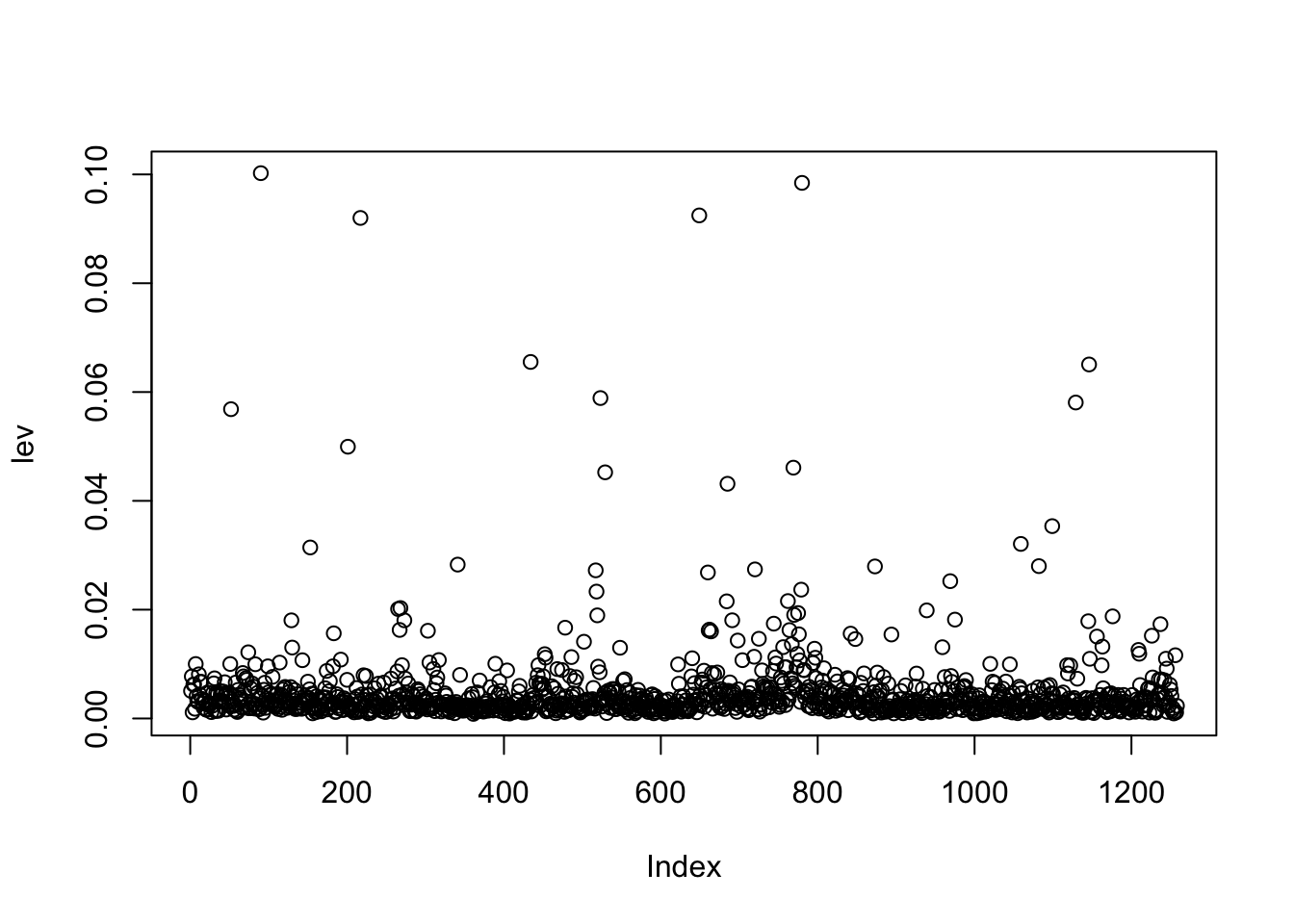where on the x-axis we have the observation index (between 1 and 1258). We want to include in the plot the threshold:

# save the value of p (n. of regressors) and n
p = length(mod1$coefficients)-1 n = nrow(logret) plot(lev) abline(h=2*(p+1)/n, col="red")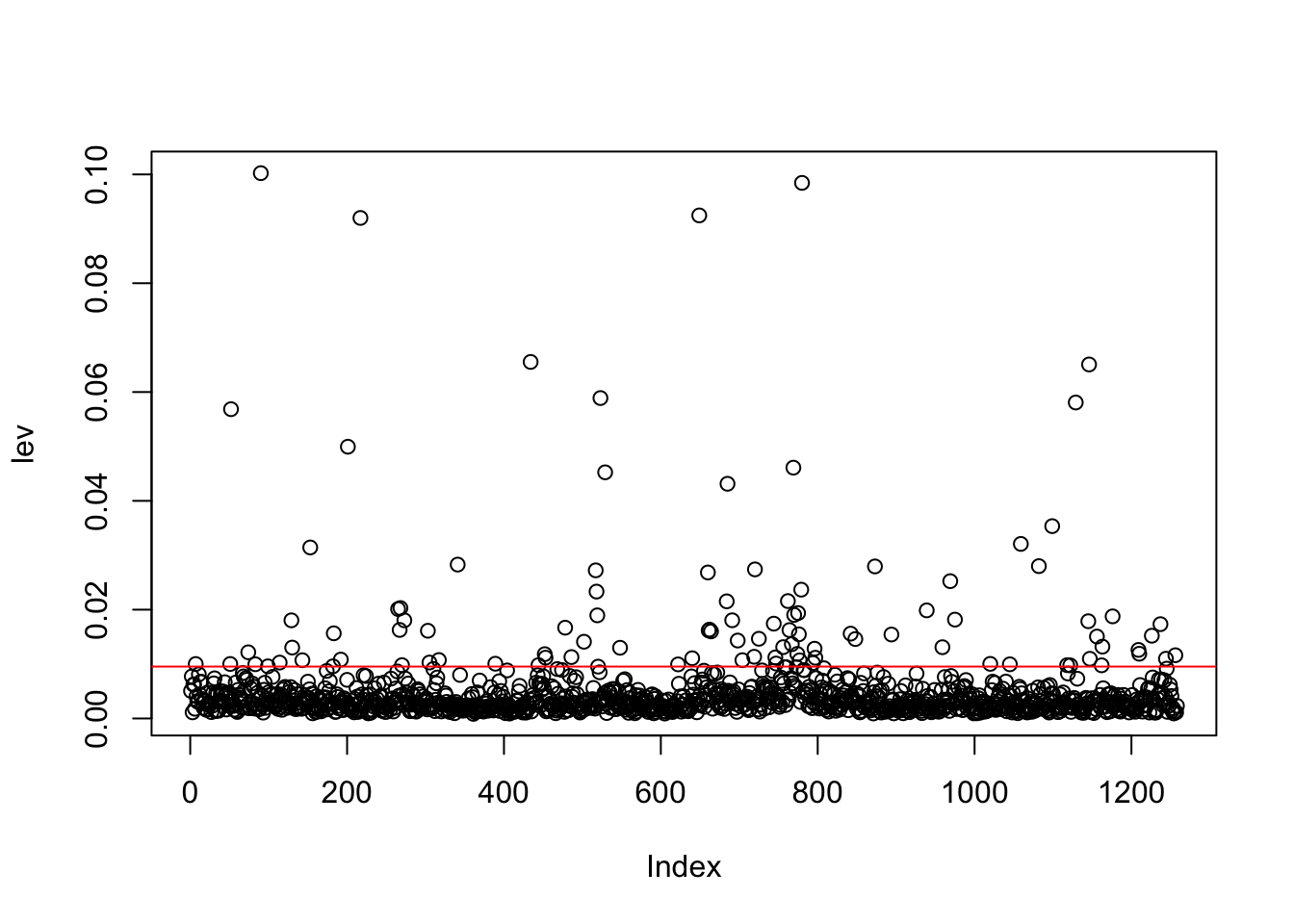To compute the number and percentage of high-leverage observations we proceed as follows: sum(lev > 2*(p+1)/n) ##  102 mean(lev > 2*(p+1)/n)*100 ##  8.108108 As represented in the bottom-left panel of Figure 11.1, having a high leverage value doesn’t mean that the observation is highly influential. ### 11.1.2 Residuals and outliers An outlier is an observation with an extreme value for the dependent variable and thus a high residual value. There are 3 different types of residuals (see also theory lectures for more details): • raw residuals: $$\hat \epsilon_i=y_i-\hat y_i$$, obtained in R with mod1$residuals.

• standardized residuals: $$\frac{\hat\epsilon_i}{\hat\sigma_\epsilon\sqrt{1-H_{ii}}}$$, given in R by rstandard(model=...).

• studentized residuals: $$\frac{\hat\epsilon_i}{\hat\sigma_{\epsilon,_{-i}}\sqrt{1-H_{ii}}}$$, given by rstudent(model=...).

We compute the studentized residuals for the considered model:

res = rstudent(mod1)

The corresponding plots are obtained by

plot(fitted(mod1),res)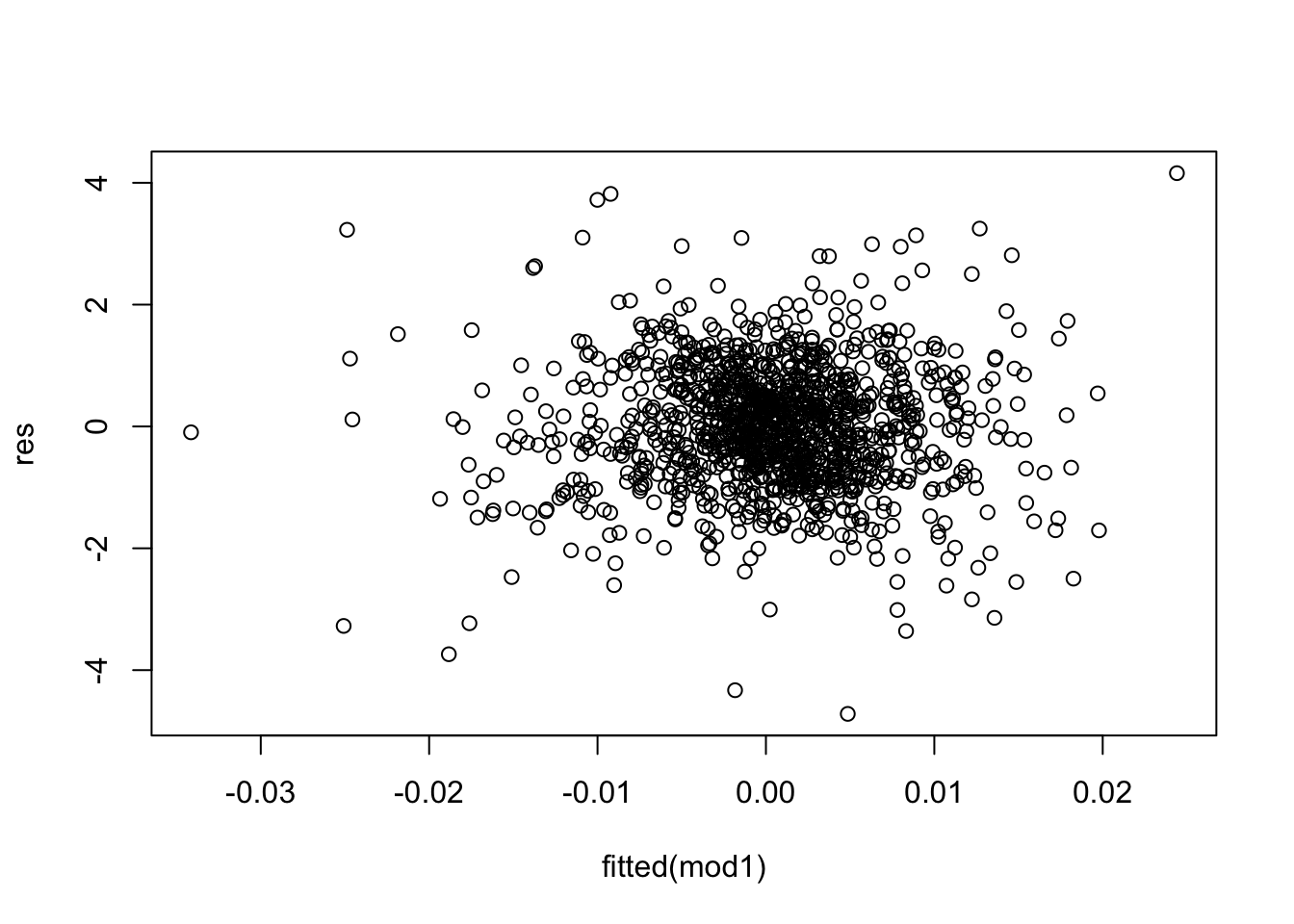hist(res)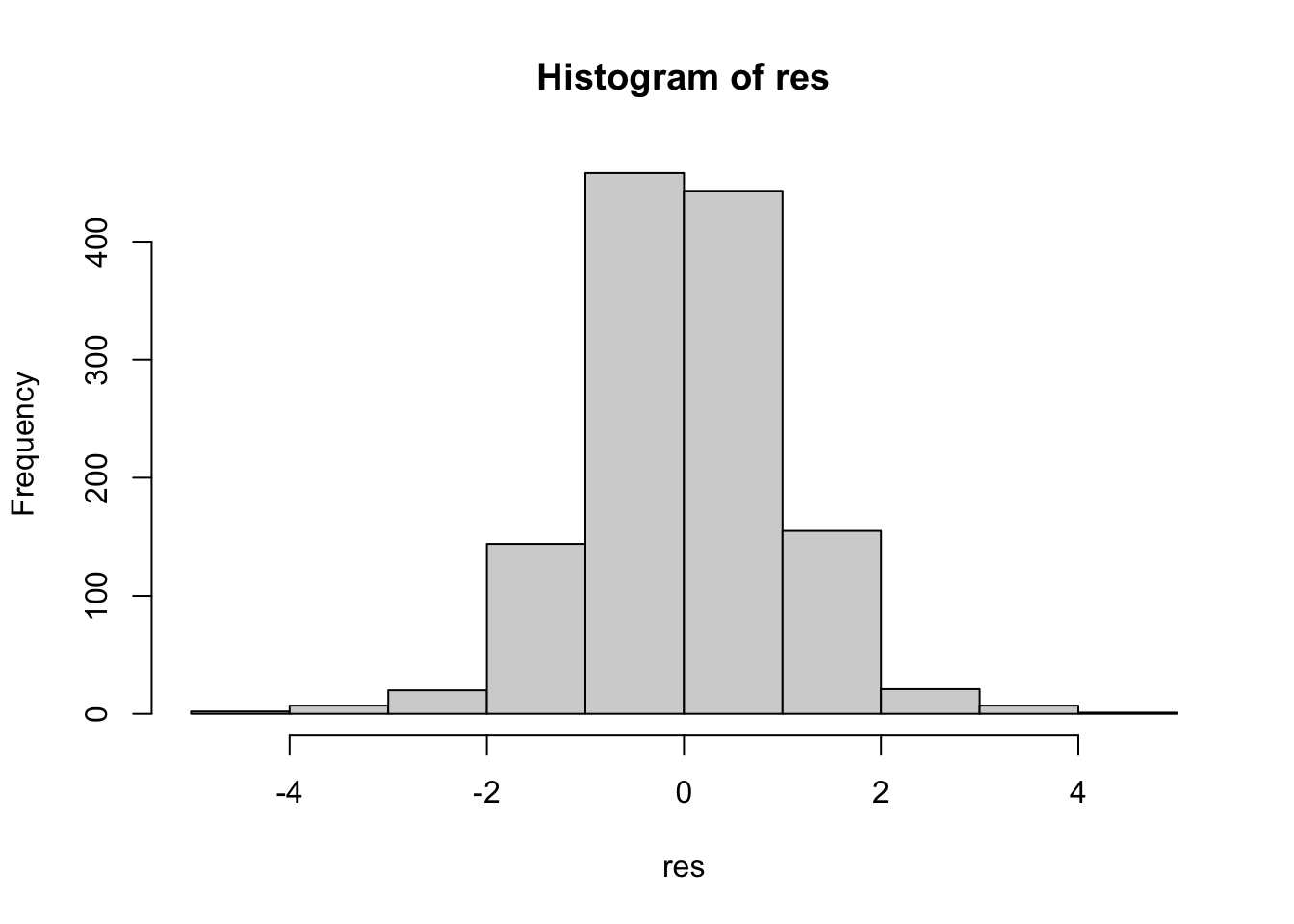The thresholds for computing the numbers of outliers (i.e. observations with a high residual) are given by -3 and +3 (or -2 and +2). We include these thresholds in the plots:

plot(res)
abline(h=c(-3,+3),col="red")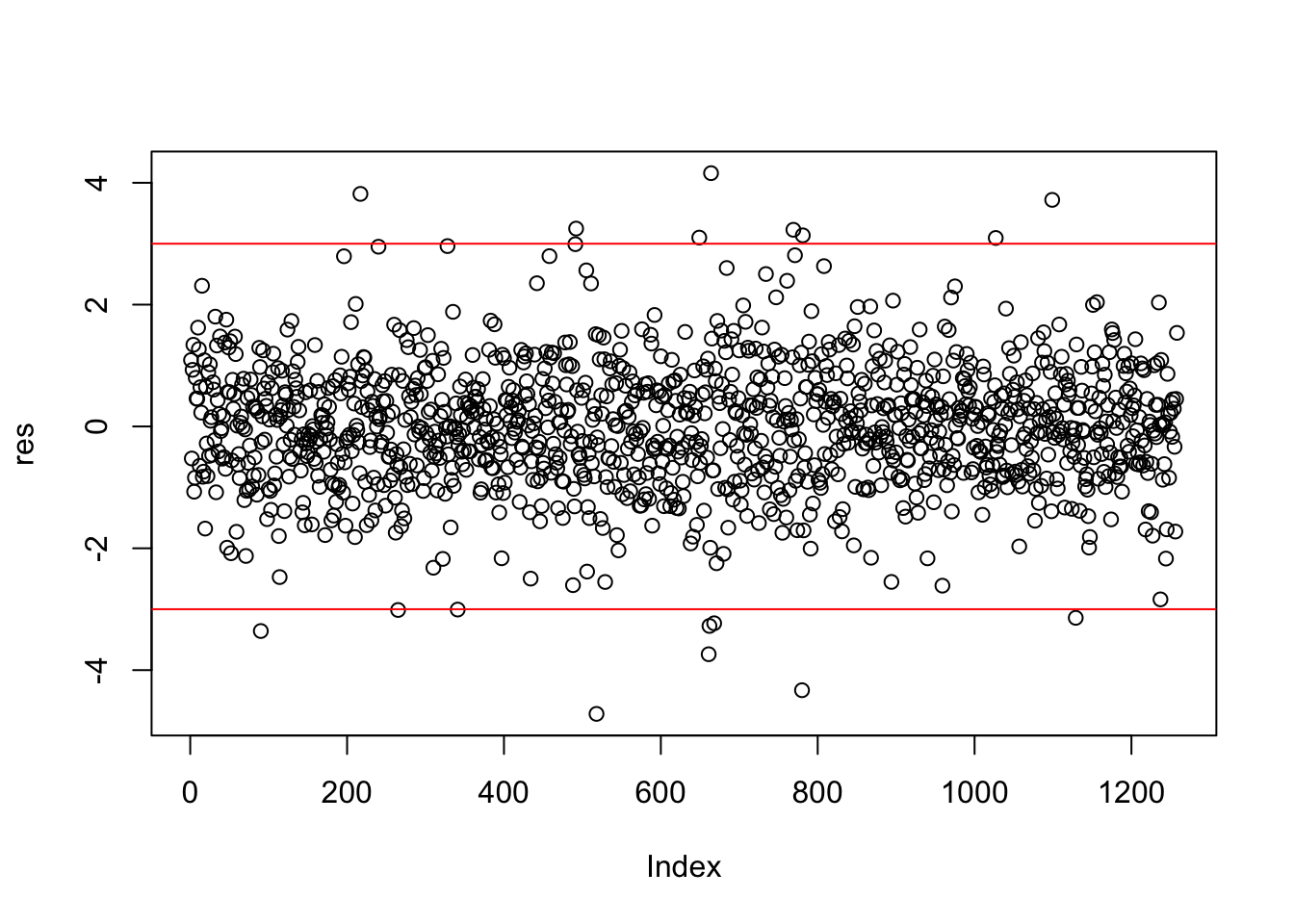hist(res)
abline(v=c(-3,+3),col="red")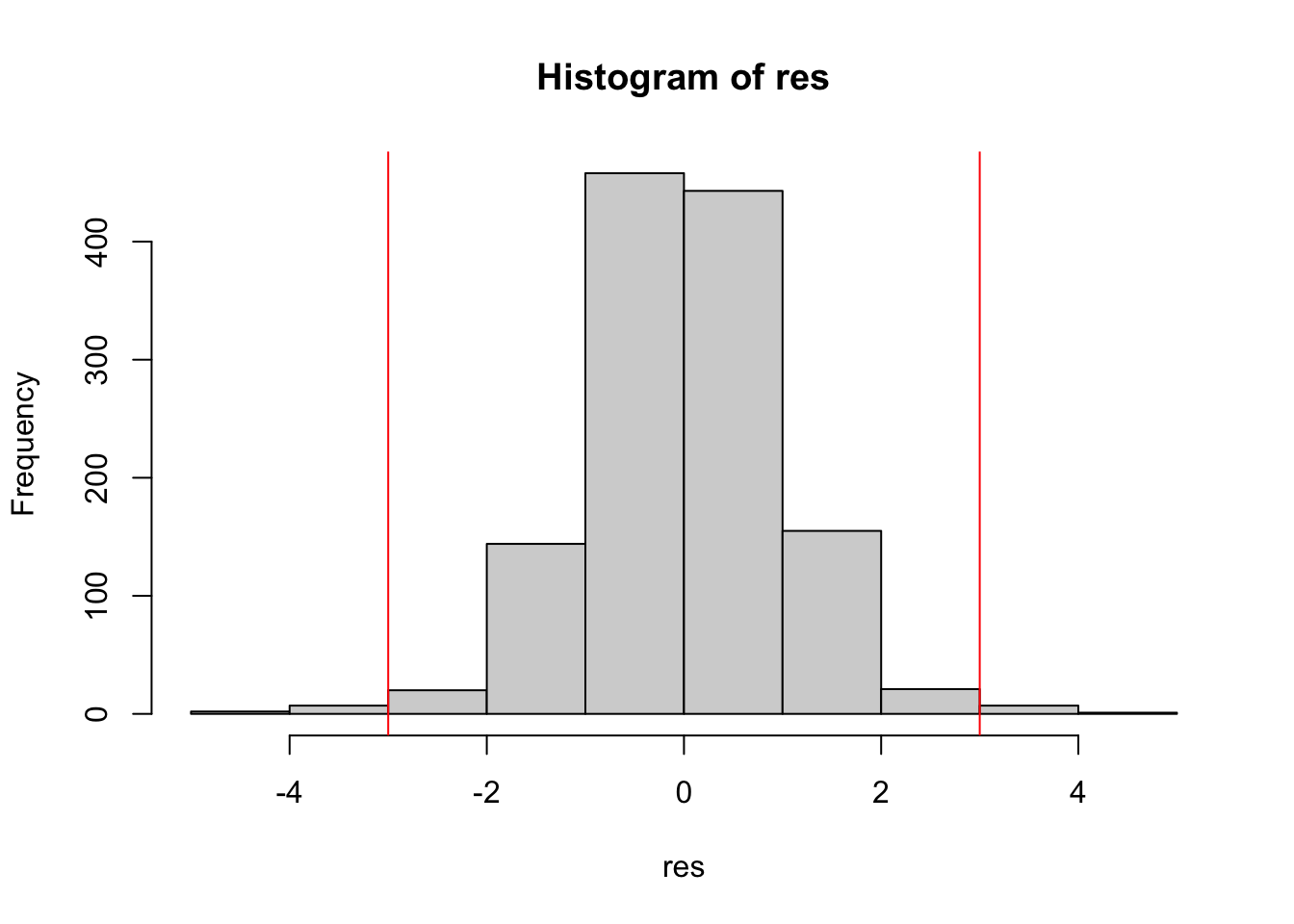The corresponding computation of the percentage of outliers is given by

mean(res < -3 | res >+3)*100
##  1.351351

The symbol | represents the OR operator. Note that in the previous code it is important to separate < from - with an empty space, otherwise <- will be interpreted as the assignment operator (and it’s not what we want…).

As represented in the top-left panel of Figure 11.1, having a high residual value doesn’t mean that the observation is highly influential.

### 11.1.3 Cook’s distance

An influential point is an observation which is able to modify the estimates of the regression line. This is given by the Cook’s distance which measures how much the predicted value $$\hat y_i$$ changes (due to the change in the slope) when the $$i$$-the observation is removed from the regression model. The Cook’s distance is computed in R by cooks.distance(model=...). The threshold is $$4/n$$.

cookdist = cooks.distance(mod1)

The plot, including the threshold, can be obtained by using the following code:

plot(cookdist)
abline(h=4/n, col="red",lwd=2)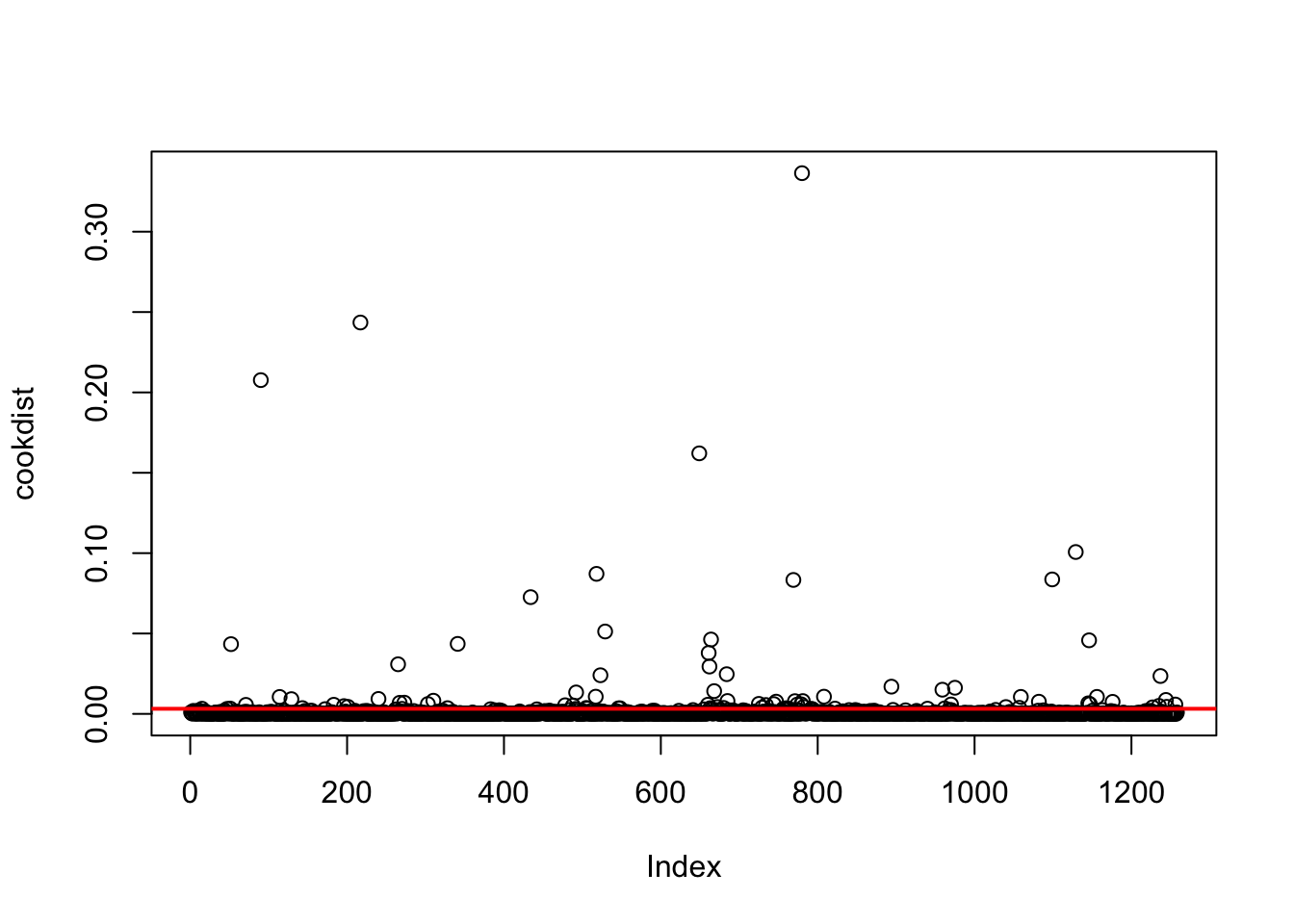And the percentage of highly influential observations is given by

mean(cookdist > 4/n)*100
##  6.518283

It is always important to investigate why we have influential observations in our dataset: are they due to mistakes in the data or to extraordinary events?

### 11.1.4 A single plot for leverage, residuals and Cook’s distance

The function influencePlot included in the library car can be used to represent the three important variables (leverage, residuals and Cook’s distance) in a single plot:

library(car) #load the library
influencePlot(mod1)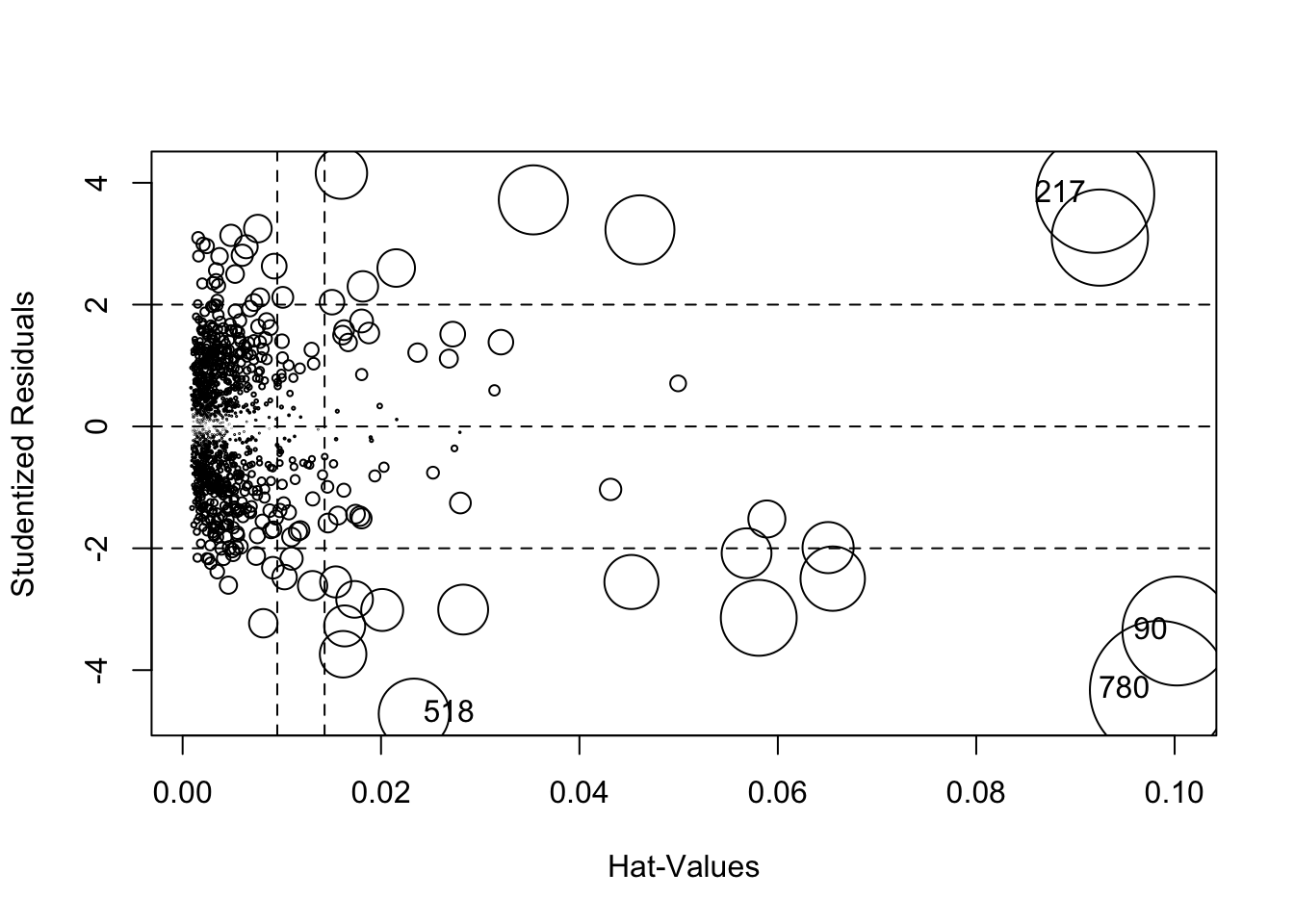##       StudRes        Hat      CookD
## 90  -3.358004 0.10022960 0.20764693
## 217  3.817804 0.09199915 0.24349483
## 518 -4.718118 0.02332257 0.08711613
## 780 -4.329769 0.09844534 0.33640968

As described in the help page of the function This function creates a “bubble” plot of Studentized residuals versus hat values, with the areas of the circles representing the observations proportional to the value Cook’s distance. Vertical reference lines are drawn at twice and three times the average hat value, horizontal reference lines at -2, 0, and 2 on the Studentized-residual scale. The function labels the points with large Studentized residuals, hat-values or Cook’s distances. In this case 4 observations are labeled and they represent the most problematic observations in the data frame. The values of the three indexes for these observations are reported in the console.

## 11.2 Residual diagnostics

After the model has been fit a residual analysis should be carried out in order to detect if there are any problems regarding the assumptions behind regression (see theory lectures). In particular we assume:

• constant variance of the errors, also known as homoschedasticity ($$Var(\epsilon_i)=\sigma^2_\epsilon$$);
• linearity of the effects of the predictor variables on the response;
• normality of the errors;
• uncorrelation of the errors, i.e. $$Cov(\epsilon_i,\epsilon_j)=0$$ for each $$i,j$$.

### 11.2.1 Homoschedasticity?

Non constant variance is also called heteroschedasticity. It means that the variability of the errors, and consequently of the response variable, is not constant. In Figure 11.2 we have two scatterplots: in the left plot it is clear that the variability of the dependent variable increases with the values of the regressor $$x$$; in this case the cloud of point has a funnel shaper. In the right plot the variability is constant across the range of $$x$$ (homoschedasticity).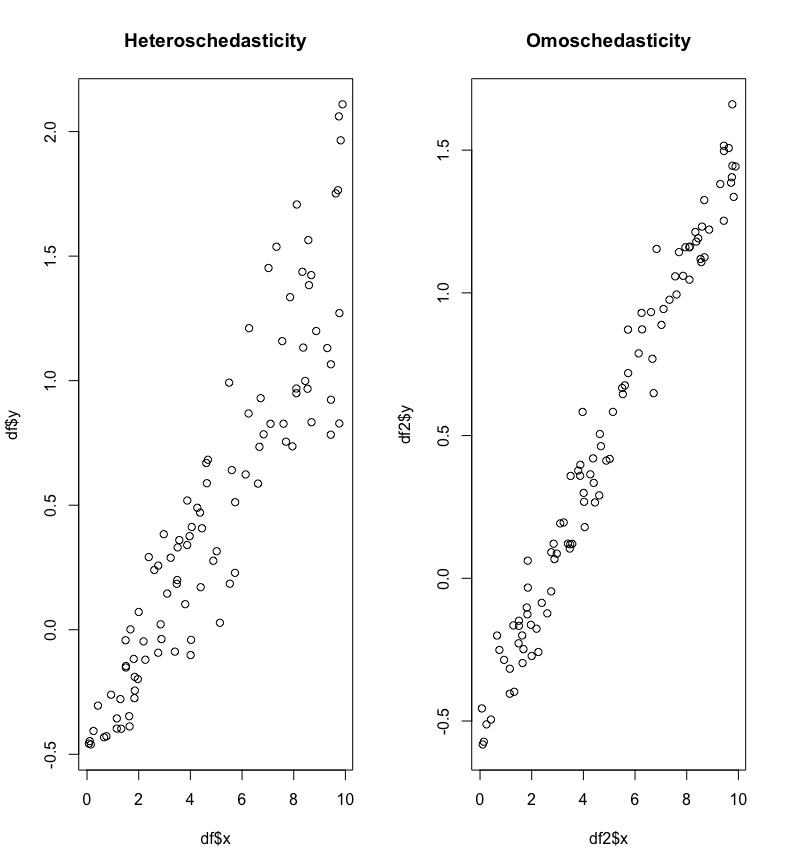Figure 11.2: Scatterplot in case of non constant and constant variance

Non constant variance can be detected by plotting the absolute (studentized) residuals against the predicted values ($$\hat y$$). A systematic trend, as the one shown in the left plot of Figure 11.3, is an indication of heteroschedasticity.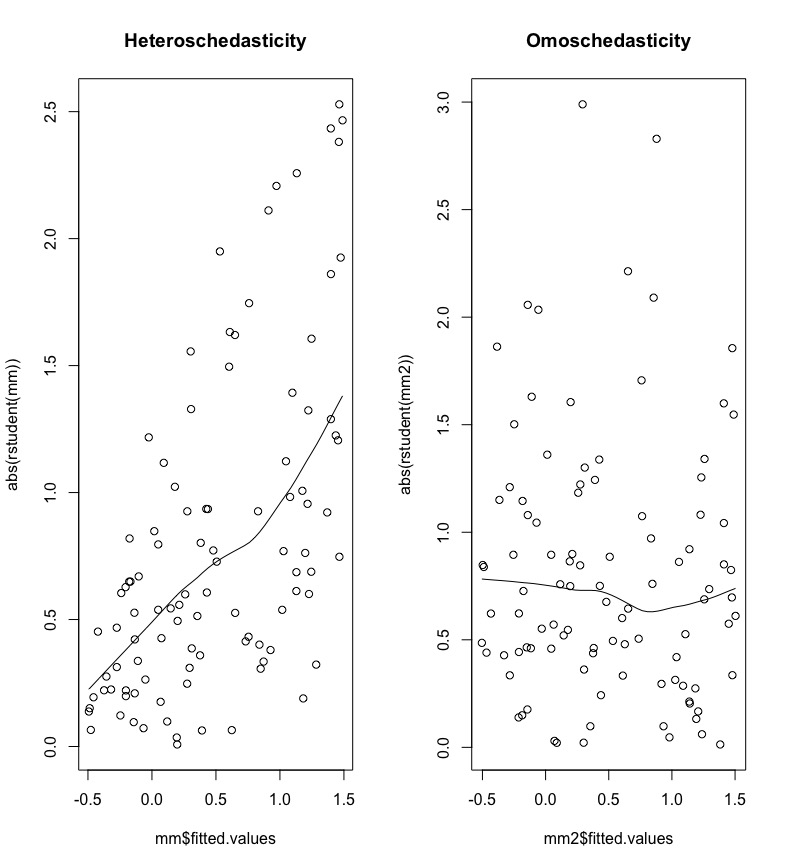Figure 11.3: Plot of absolute (studentized) residuals against predicted values.

For the data under analysis we obtain the following plot:

scatter.smooth(mod1$fitted.values,abs(res))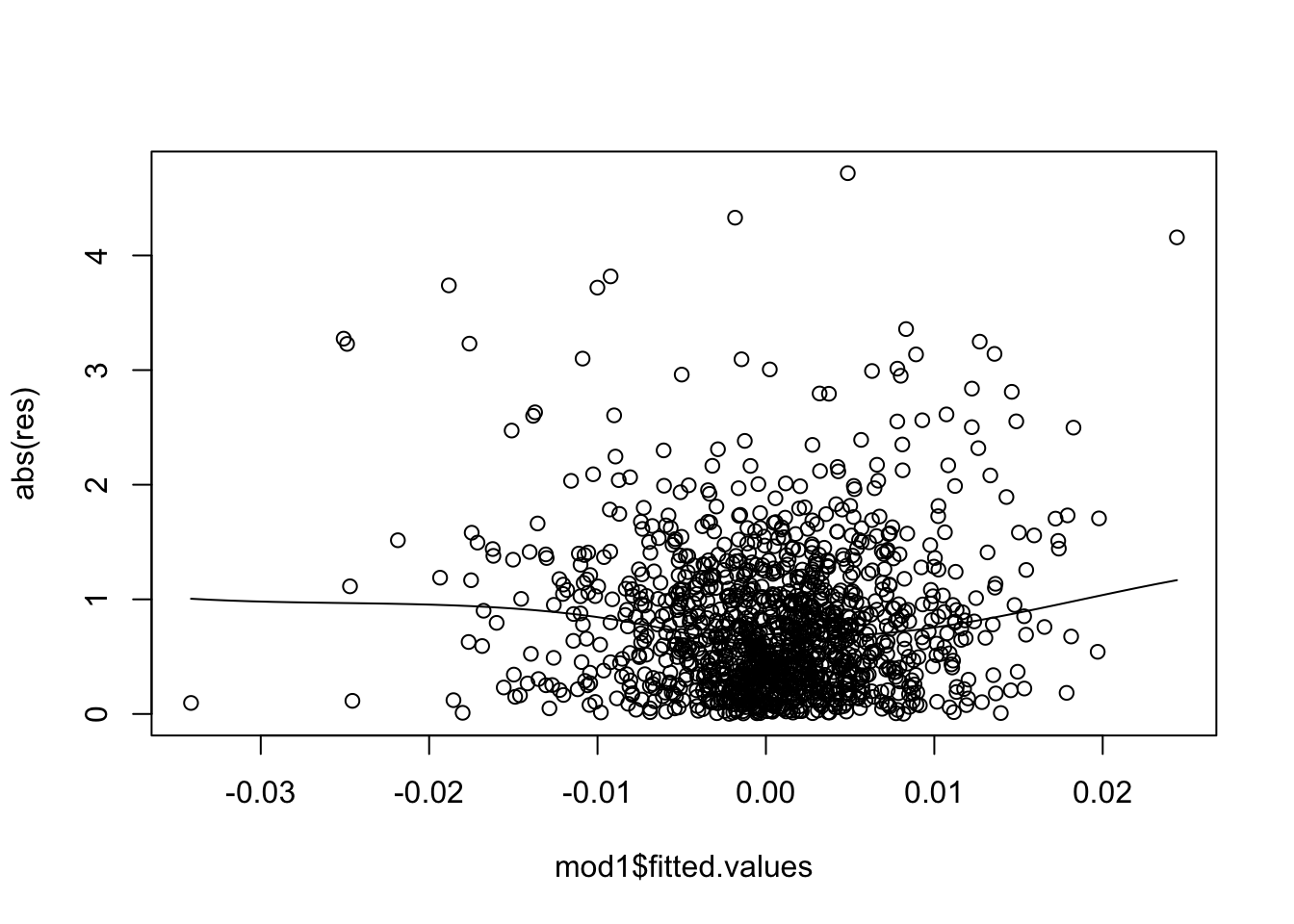Note that we use the function scatter.smooth instead of plot for including in the plot a smooth line that can be considered as a summary of the cloud of points and can ease the interpretation. The plot shows no evident trend and we can conclude that the assumption of homoschedasticity can be accepted. ### 11.2.2 Linearity? The definition of the linear regression model assumes that the predictor variables have a linear effect on the response. In the left plot of Figure 11.4 we observe a non linear relationship between $$x$$ and $$y$$ (it seems more like a quadratic relationship).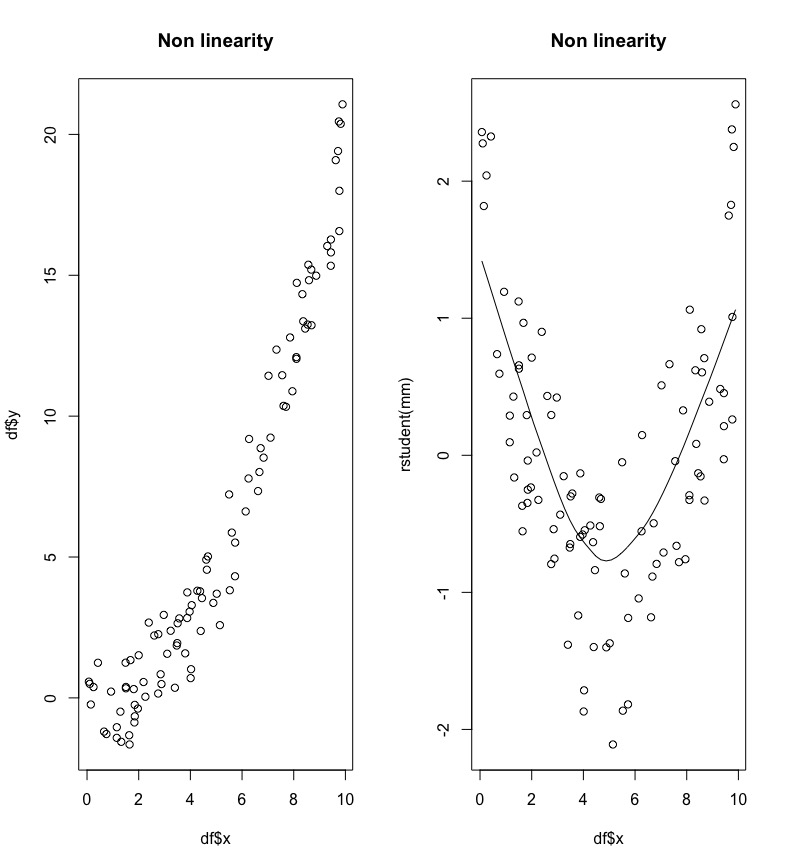Figure 11.4: A case of non linearity between x and y. Non linearity can be detected by plotting residuals against the value of each predictor, as shown in the right plot of Figure 11.4; a non linear pattern is an indication of non linearity. For the considered case we obtain the following 5 plots: scatter.smooth(logret$BA,res)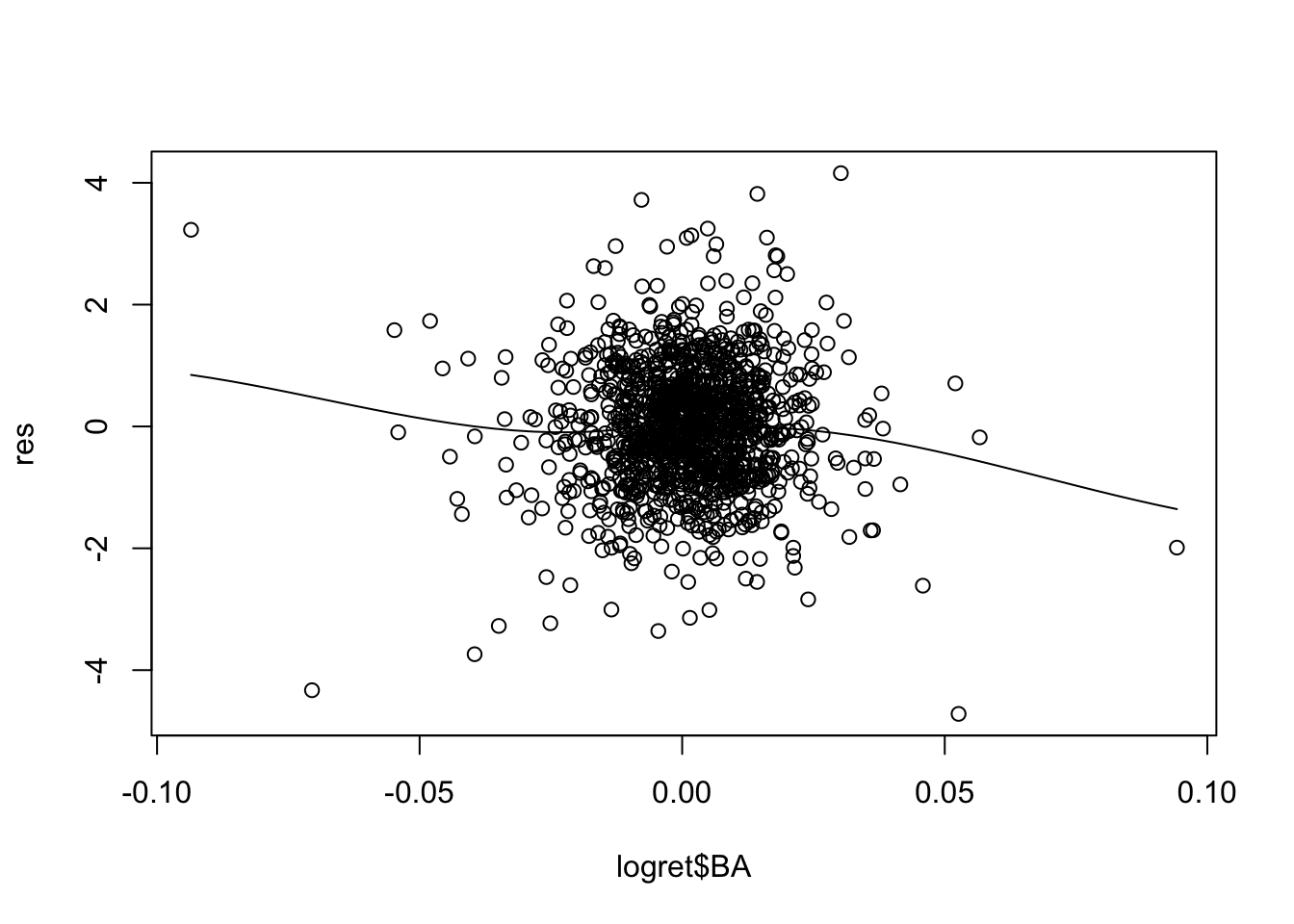scatter.smooth(logret$CAT,res)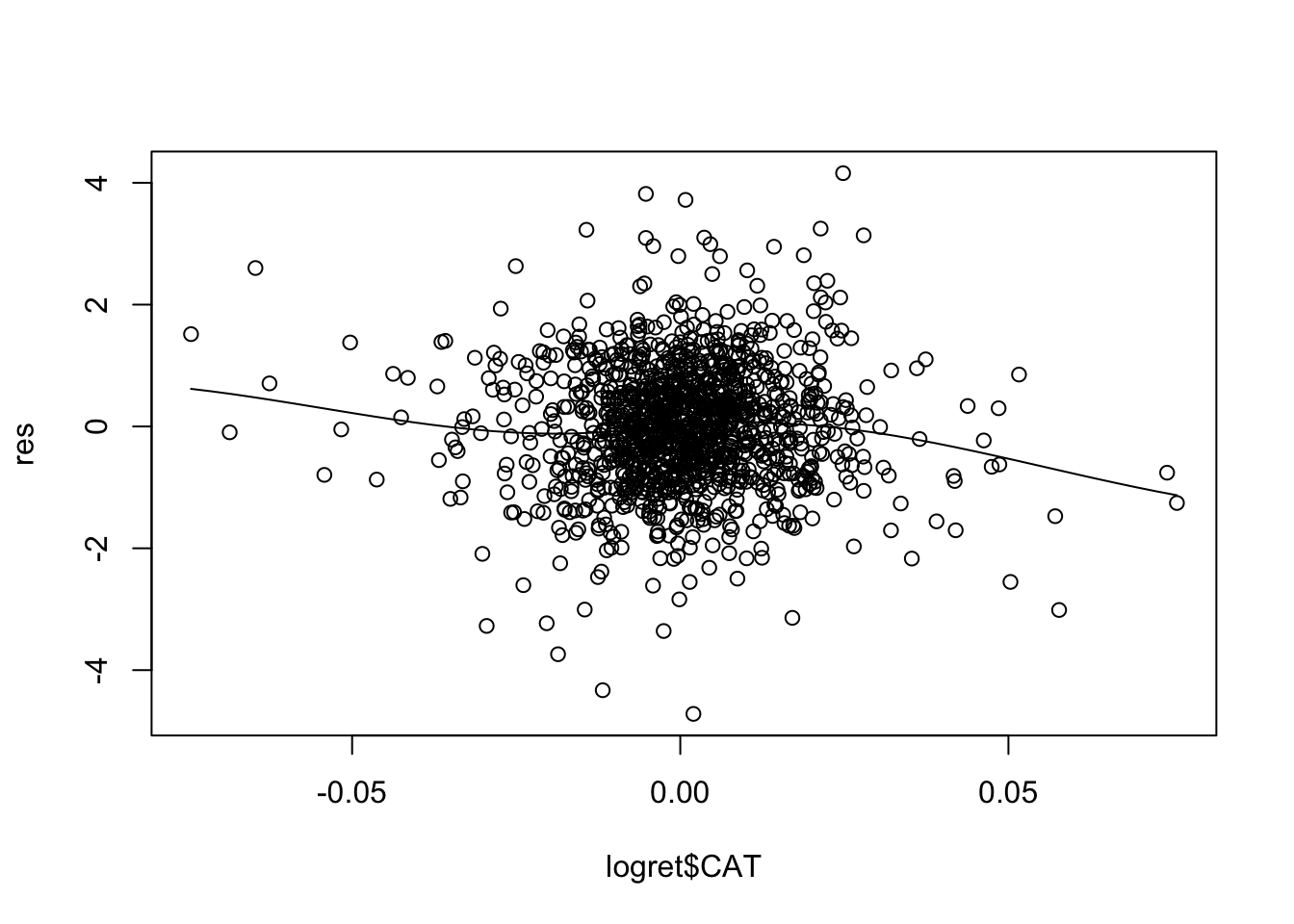scatter.smooth(logret$CSCO,res)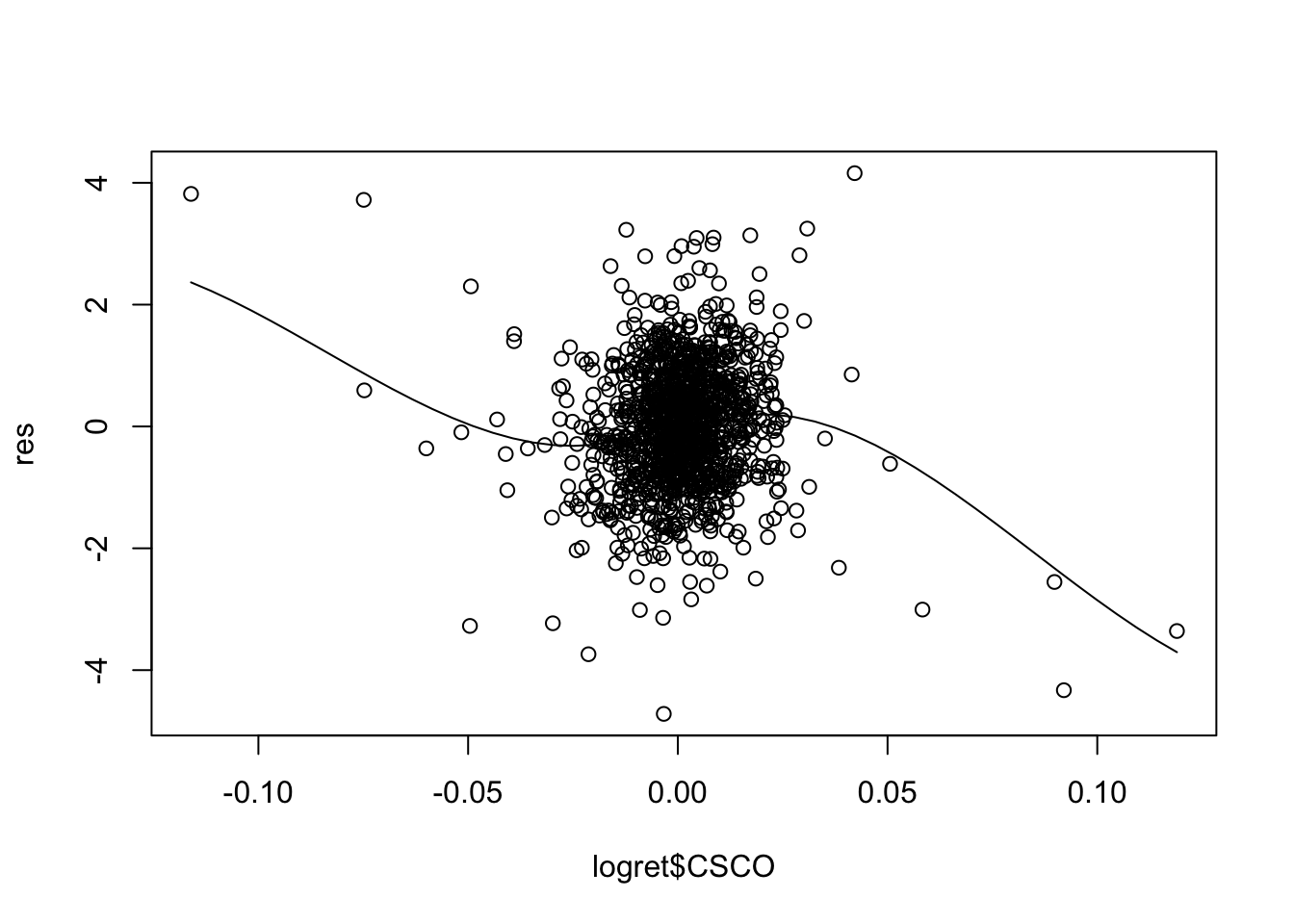scatter.smooth(logret$NKE,res)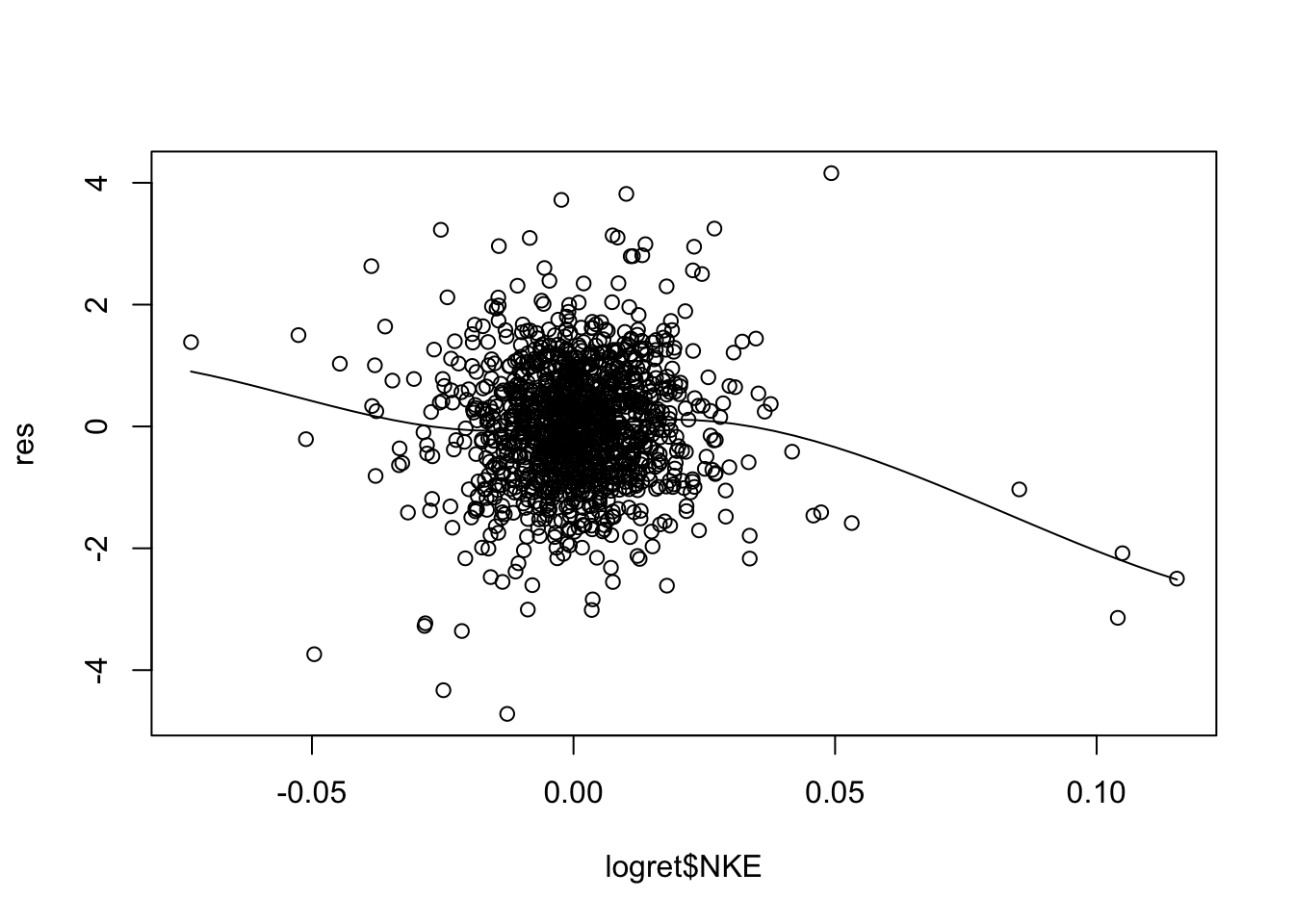scatter.smooth(logret$DIS,res)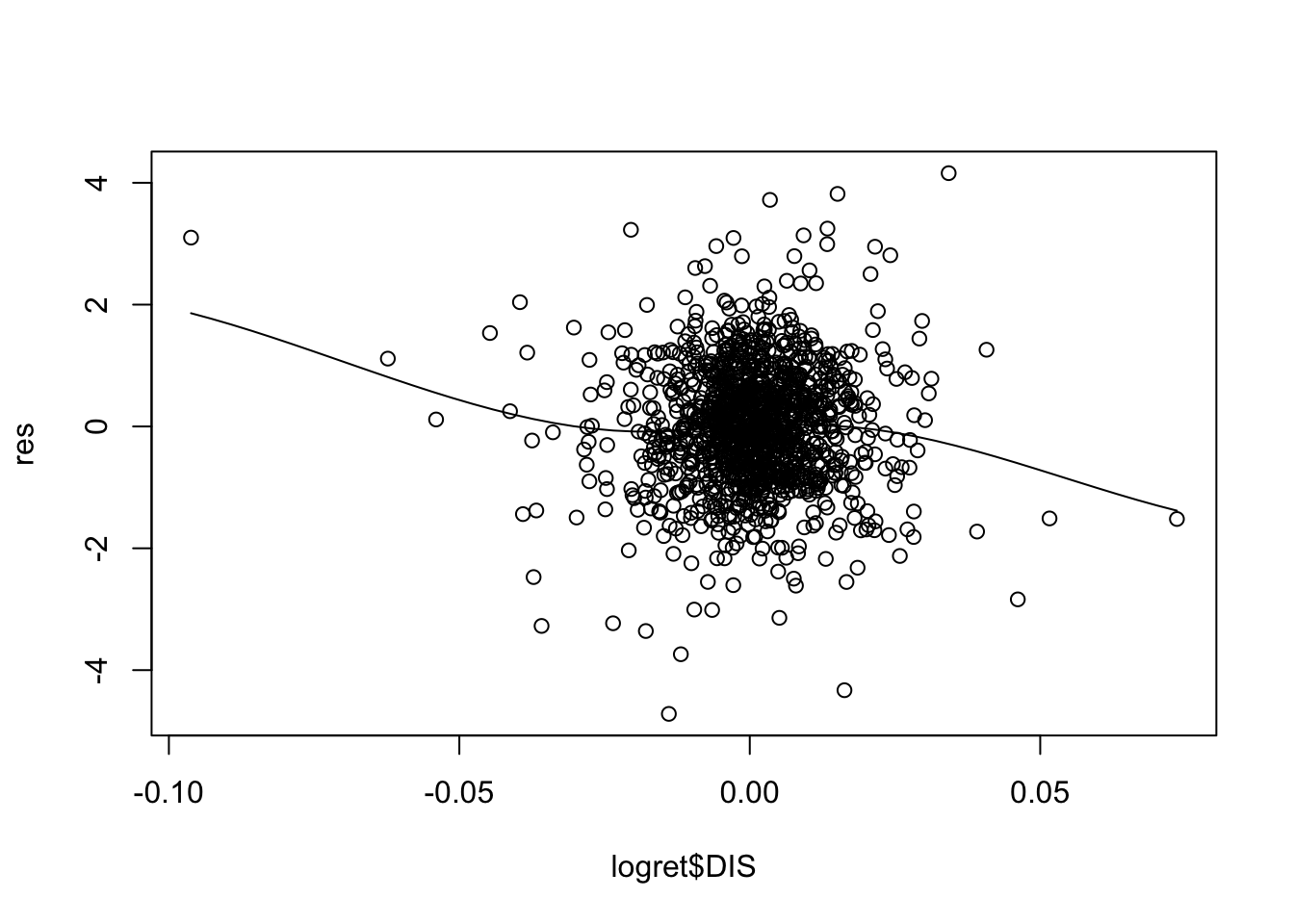For some of the regressors the smooth line is showing a non linear pattern (especially for CSCO). A possible solution is to add, for example, $$X^2$$ and possibly $$X^3$$ in the set of regressors (in this case we speak about polynomial regression).

### 11.2.3 Uncorrelation?

To check if it exists some correlations in the residuals we use the correlogram, a graphical representation that is used extensively for time series analysis. It can be obtained by using the acf (autocorrelation) function.

acf(res)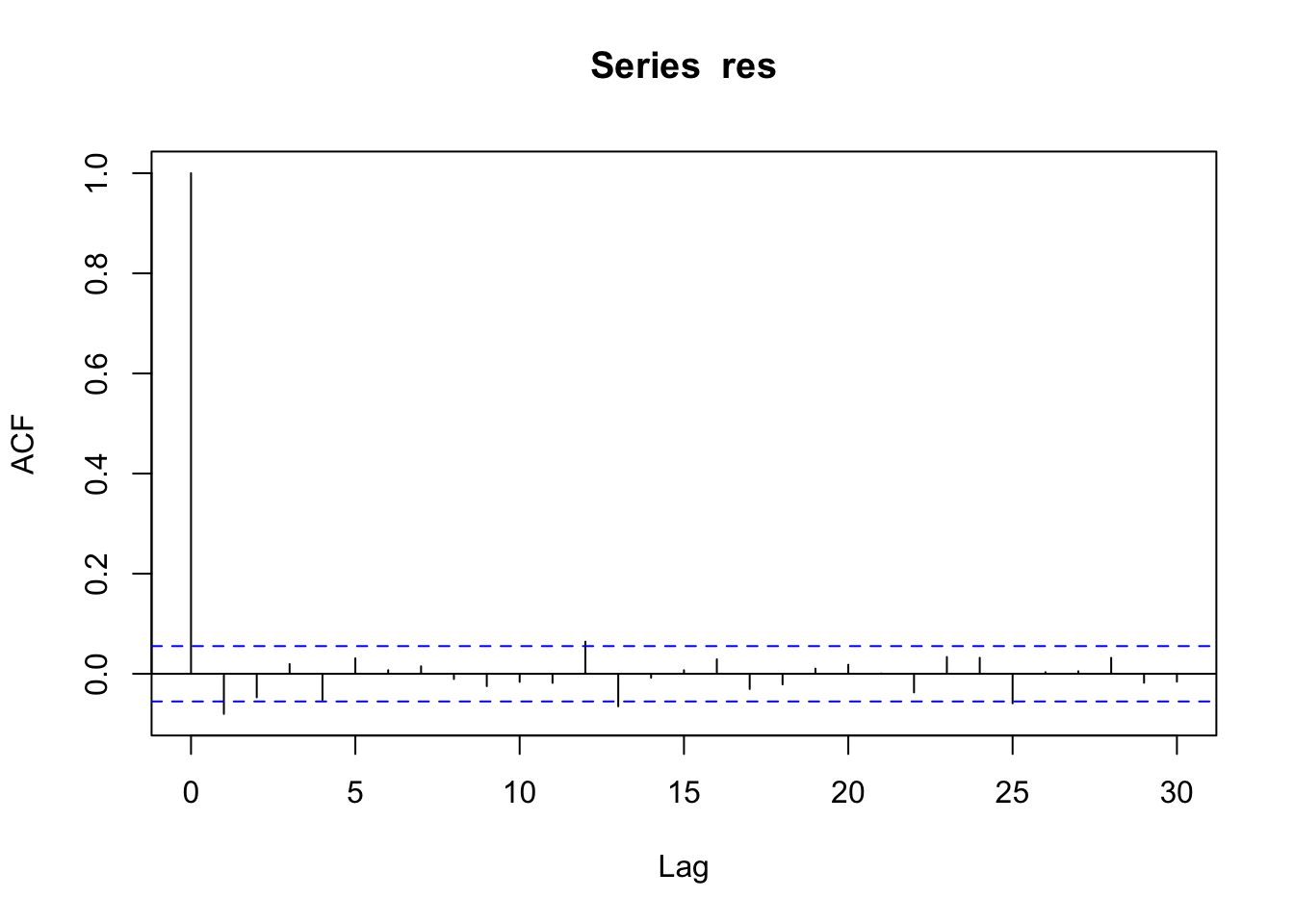The correlogram has lags (time distance) on the x-axis and the value of the autocorrelation on the y-axis. The first bar is always equal to one because it corresponds to $$Cor(y_t,y_t)$$ with $$t=1,\ldots,n$$. The second vertical bar for $$h=1$$ is negative and significantly different from zero (as it is outside the blue horizontal lines). Thus the lag-one correlation $$Cor(y_t,y_{t-1})$$ is significant. This leads to the conclusion that the residuals are not uncorrelated. This suggests to use a specific model for correlated data (e.g. ARIMA models).

### 11.2.4 Normality?

To check the normality assumptions we use the tools introduced in the previous labs, such as for example the normal probability plot:

qqnorm(res,datax = T)
qqline(res,datax = T)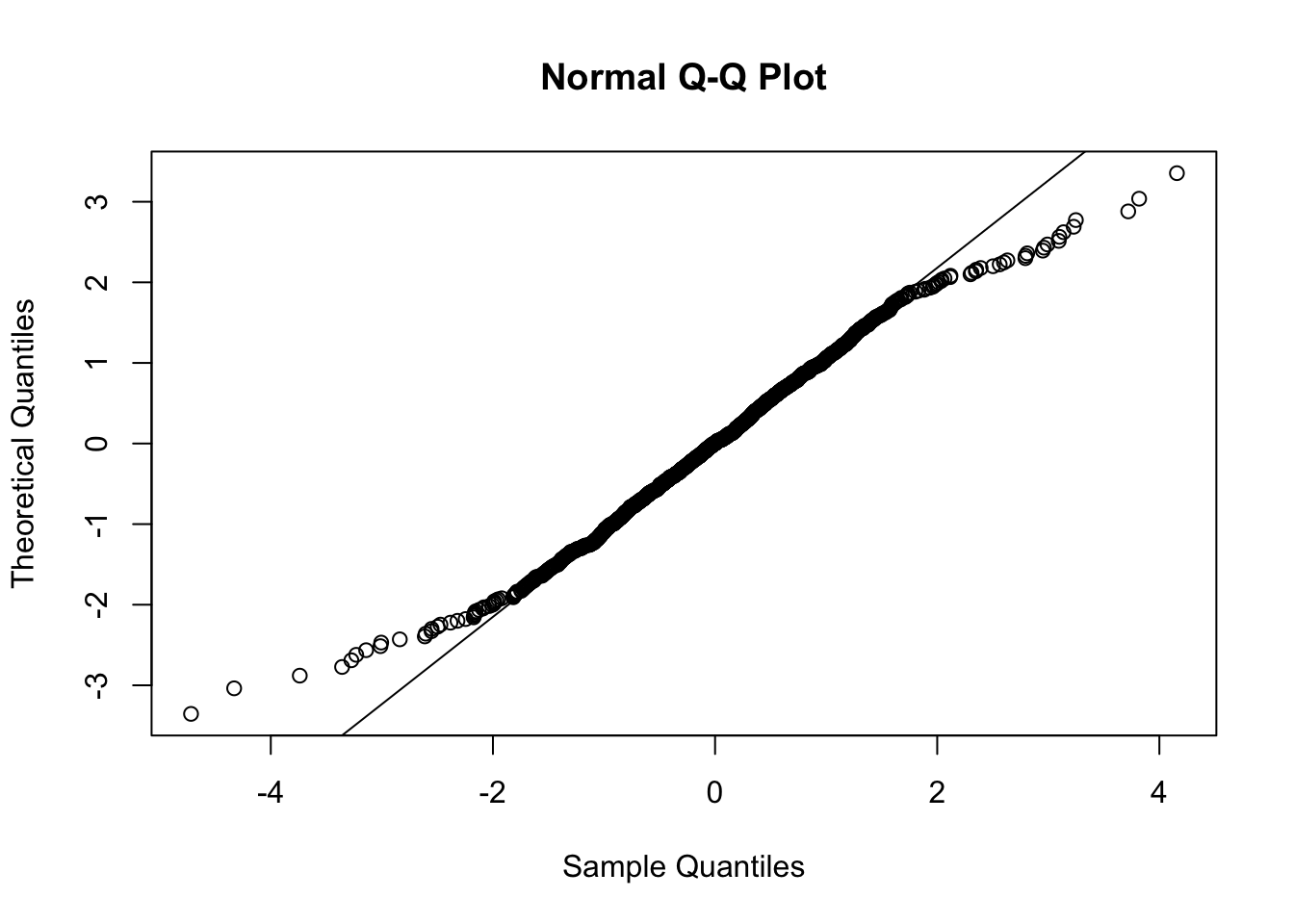It is characterized by a convex-concave pattern which is typical of distributions with tails heavier than the normal distribution. We also perform a test to check the normality condition:

shapiro.test(res)
##
##  Shapiro-Wilk normality test
##
## data:  res
## W = 0.98784, p-value = 9.853e-09

Given the value of the p-value we reject the normality assumption.

## 11.3 Exercise Lab 9

### 11.3.1 Exercise 01

The file “returns_GrA.csv” contains the daily log returns for the following indexes/assets: Dow Jones Industrial Average (DJI), NASDAQ Composite (IXIC), S&P 500 (GSPC) and Visa Inc. (V), from 21-11-2012 to 20-11-2017.

The file “GS.csv” contains the daily prices and volumes for The Goldman Sachs Group, Inc. (GS) asset (from 20-11-2012 to 20-11-2017).

Import the two datasets in R and create two objects named returnslog and GS.

1. Compute the series of log returns for the GS asset (use the Adj.Close prices) and add it as a new column of returnslog.
• Provide the plot containing the 5 boxplots (placed side by side). Moreover, add to the boxplot a horizontal line for the values -0.05 and +0.05.

• Comment the plot.

• Compute the percentage of days with log returns bigger than 0.05 OR lower than -0.05.

1. Compute the correlation matrix for the available log returns time series. Comment the values.

2. Estimate a multiple regression model with the log returns of Visa Inc. (V) as dependent variable and with all the other log returns variables (DJI, IXIC, GSOC and GS) as regressors (full model).

• Provide the full model summary.

• Discuss the significance of the model coefficients (if needed, use $$\alpha$$ = 0.01).

• Compute the goodness of fit index by using the formula $$R^2=1-SSE/SST$$.

• Compute the goodness of fit index by using the formula $$R^2=Cor(y,\hat y)$$^2.

1. Discuss and compare the goodness of fit index of the full model (obtained at point 3.) and of the reduced model (after removing the non significant regressors). Provide also the reduced model’s summary. What can you conclude, if you have to choose between the full and the reduced model?

2. For the model chosen at the previous point:

• plot the Cook’s distance (y axis) and leverage (x axis) values and add to the plot the thresholds for detecting high leverage/influence points.

• How many points (days) with high leverage and high influence do we have (in %)?

• Consider the day with the highest leverage. On which day did it occur?

• Study the studentized residuals in order to check if the model assumptions are met.

### 11.3.2 Exercise 02

Consider the file simdata.csv containing simulated values for a response variable y and 2 covariates (x1 and x2) for $$n=500$$ observations. Import the data in R.

1. Plot the response variable. What can you conclude?

2. Implement the full regression model.

• Provide and comment the summary output.

• Consider the studentized residuals and check the assumptions of the regression model. What can you conclude? Which problems do you observe?

1. Using the Box-Cox transformation transform the response variable to solve the skewness problem (add the transformed variable to the dataframe).
• Plot the transformed variable.

• Implement again the full regression model. Provide and comment the summary output.

• Consider the studentized residuals and check the assumption of the regression model.

• Consider a non linear effect of $$X_1$$ in your model equation: $$Y = \beta_0+\beta_1X_1+\beta_2X_1^2+\beta_3X_2+\epsilon$$. You will have to use the following formula in R: y ~ poly(x1,degree=2,raw = F)+x2.

• Provide and comment the summary output.

• Consider the studentized residuals and check the assumption of the regression model.